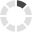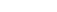### Site InformationLoading... Please wait...

# MAT 543 MAT543 MAT/543 Exam 2 (Strayer 2020)

\$16.99

## Product Description

Strayer MAT 543 Exam 2 Answers - 2020

1. What should be the goal of analytic forecast methods?
2. What is analytic forecasting useful for predicting?
3. A manager who is effective with forecasting will be able to do what?
4. Most forecasting problems faced by the health services manager can be accomplished using some form of forecasting known as what?
5. Which assumption would guide decision making when attempting to forecast the impact of a new government regulation?
6. Which moving average best minimizes the variation in historical data and is the recommended moving average to use for forecasting?
7. If forecasting using 33 months of historical data, how many months should you forecast if using exponential smoothing, SC=.3?
8. Which is one of the three guiding principles of future forecasting?
9. Using moving averages for n = 2 and n = 3 will produce what types of forecasts?
10. Average percent change as a forecasting method relies on what primary assumption?
11. What does the y-intercept of a regression equation represent?
12. What do regression lines look like?
13. What does a slope of zero mean?
14. What is the fundamental mathematical principal behind regression?
15. Which of the following is the null hypothesis used to test whether a regression line may or may not be used?
16. An airplane with 100 seats can fly no more than 100 passengers at any one time.  The limit of a system’s finite capacity is called what?
17. What is true of system capacity?
18. How can the capacity of any specific work process be determined?
19. Capacity analysis provides a quantitative methodology for establishing what?
20. The price per test is \$85, and the variable cost per test is \$15.  Using this information, what is the breakeven point in volume if the allocated fixed costs were \$350,000?
21. In the language of queuing theory, what occurs when a unit waiting in line for service decides that the wait is too long and leaves the line?
22. Queuing theory calculations describe arrivals for the line according to which probability distribution?
23. What is it called when a unit switches lines in order to get on a shorter line?
24. In a single channel single server system, if the service rate is 4 per hour and the arrival rate is 6 per hours, what is the probability that a unit must wait (Pw)?
25. When queuing theory models are used in the development of appointment system, giving appointments every 15 minutes is the same as an arrival rate of how many per hour?

## Customers also viewed

• \$16.99• \$16.99• \$13.99• \$7.99• \$7.99## Related Products

Click the button below to add the MAT 543 MAT543 MAT/543 Exam 2 (Strayer 2020) to your wish list.